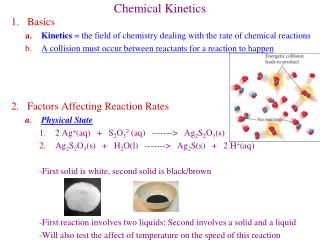DownloadDownload PresentationChemical Kinetics

Chemical Kinetics

Download PresentationChemical Kinetics

- - - - - - - - - - - - - - - - - - - - - - - - - - - E N D - - - - - - - - - - - - - - - - - - - - - - - - - - -
Presentation Transcript

1. Chemical Kinetics • Basics • Kinetics = the field of chemistry dealing with the rate of chemical reactions • A collision must occur between reactants for a reaction to happen • Factors Affecting Reaction Rates • Physical State • 2 Ag+(aq) + S2O32-(aq) -------> Ag2S2O3(s) • Ag2S2O3(s) + H2O(l) -------> Ag2S(s) + 2 H+(aq) -First solid is white, second solid is black/brown -First reaction involves two liquids; Second involves a solid and a liquid -Will also test the affect of temperature on the speed of this reaction

2. Concentration • Mg(s) + 2 HCl(aq) -------> MgCl2(aq) + H2(g) • DO THIS REACTION IN THE HOOD; H2(g) is flammable • We will be making 5 different concentrations of HCl 2 ml 1.0 M HCl + 8 ml H2O = 0.2 M HCl 4 ml 1.0 M HCl + 6 ml H2O = 0.4 M HCl etc...

3. Surface Area • Zn(s) + 2 HCl(aq) -------> ZnCl2(aq) + H2(g) • DO THIS REACTION IN THE HOOD; H2(g) is flammable • We will run the reaction twice, with different size Zn particles • Smaller particles have more surface area

4. d. Temperature 2MnO4-(aq) + 5C2O42-(aq) + 16H+(aq) -----> 2Mn2+(aq) + 10CO2(aq) + 8H2O(l) purple colorless • We will run the same reaction at 25 oC, 40 oC, 55 oC, and 70 oC • Room temp. will be slow, stop it after 15 min (record > 15min as time) • Catalyst • A catalyst speeds up a reaction without being used up itself • It works by lowering the Activation Energy • Mn2+ catalyzes the reaction (Autocatalysis); add some and time reaction